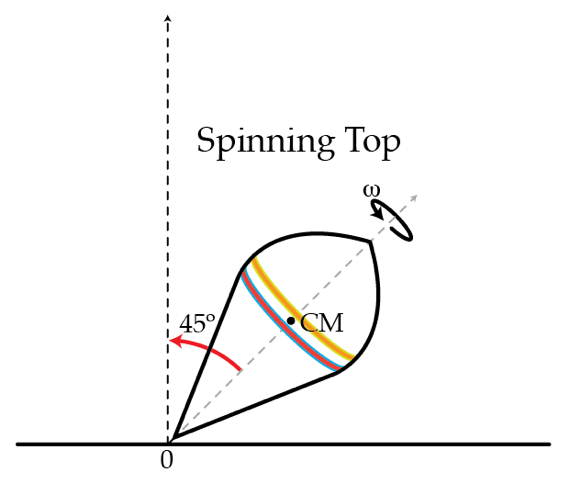# Dancing top

A top of mass $m=0.5~\mbox{kg}$ rotates with angular speed $\omega=350~\mbox{rad/s}$ around its axis which is tilted by a (constant) angle of $\theta=45^{\circ}$ as shown in the figure. The moment of inertia with respect to the top's axis is $I=2 ~\mbox{g} \cdot\mbox{m}^{2}$ and the distance from the tip of the top to the center of mass is $l=10~\mbox{cm}.$ Determine the force of friction $f$ in Newtons acting on the tip of the top.×